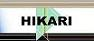| Home | Journals | Books | Paper submission | About us and our mission | News | Contact us |Site Search:

## Online Edition, Vol. 5, 2011, no. 17-20

Gao Hongya, Guo Jianling
On the integrability for the Jacobian in anisotropic Sobolev space
Int. Journal of Math. Analysis, Vol. 5, 2011, no. 17-20, 803-810.

Haihua Wang, Yanqiong Liu
Existence results for fuzzy integral equations of fractional order
Int. Journal of Math. Analysis, Vol. 5, 2011, no. 17-20, 811-818.

K. S. Sisodia, M. S. Rathore, D. Singh, S. S. Khichi
A common fixed point theorem in fuzzy metric spaces
Int. Journal of Math. Analysis, Vol. 5, 2011, no. 17-20, 819-826.

I. B. Bapna, N. Mathur
Applications of subordination on subclasses of meromorphically univalent functions with integral operator
Int. Journal of Math. Analysis, Vol. 5, 2011, no. 17-20, 827-835.

L. Kikina, K. Kikina
A related fixed point theorem in tree fuzzy metric spaces
Int. Journal of Math. Analysis, Vol. 5, 2011, no. 17-20, 837-848.

H. P. Waghamore, N. Shilpa, L. N. Achala
Uniqueness theorems sharing one value of entire and meromorphic functions
Int. Journal of Math. Analysis, Vol. 5, 2011, no. 17-20, 849-862.

Application of variational iteration method to partial differential equation systems
Int. Journal of Math. Analysis, Vol. 5, 2011, no. 17-20, 863-870.

Application of homotopy-perturbation method and variational iteration method to three dimensional diffusion problem
Int. Journal of Math. Analysis, Vol. 5, 2011, no. 17-20, 871-880.

P. Jenita, A. R. Meenakshi
Characterization of set of k-g-inverses
Int. Journal of Math. Analysis, Vol. 5, 2011, no. 17-20, 881-886.

A. El Bakkali, A. Tajmouati
On holomorphically decomposable Fredholm spectrum and commuting Riesz perturbations
Int. Journal of Math. Analysis, Vol. 5, 2011, no. 17-20, 887-900.

R. Jain, B. Bundela
A study of some incomplete elliptic integrals and associated definite integrals involving single and multivariable polynomials and functions
Int. Journal of Math. Analysis, Vol. 5, 2011, no. 17-20, 901-910.

B. Amudhambigai, M. K. Uma, E. Roja
On somewhat pairwise fuzzy open functions in smooth fuzzy bitopological spaces
Int. Journal of Math. Analysis, Vol. 5, 2011, no. 17-20, 911-922.

Bilalov B.T., Najafov T.I.
On the approximation properties of some systems in Banach spaces
Int. Journal of Math. Analysis, Vol. 5, 2011, no. 17-20, 923-933.

S. M. Mirzaei
Fredholm integral equations of the first kind solved by using the homotopy perturbation method
Int. Journal of Math. Analysis, Vol. 5, 2011, no. 17-20, 935-940.

E. Suntonsinsoungvon
The nonlinear equation of the operator \circledast_B^k related to the Bessel biharmonic equation
Int. Journal of Math. Analysis, Vol. 5, 2011, no. 17-20, 941-952.

New exact analytic solutions for stable and unstable nonlinear Schrodinger equations with a cubic nonlinearity
Int. Journal of Math. Analysis, Vol. 5, 2011, no. 17-20, 953-974.

Y. Choo, G. K. Choi
On the zero-free regions of polynomials
Int. Journal of Math. Analysis, Vol. 5, 2011, no. 17-20, 975-981.

Y. Choo
Further generalizations of Enestrom-Kakeya theorem
Int. Journal of Math. Analysis, Vol. 5, 2011, no. 17-20, 983-995.

A. Beitollahi, P. Azhdari
\varphi − H contraction in fuzzy metric space
Int. Journal of Math. Analysis, Vol. 5, 2011, no. 17-20, 997-1001.

B. Rebiai
Global classical solutions for coupled reaction-diffusion systems without growth conditions on the nonlinearities
Int. Journal of Math. Analysis, Vol. 5, 2011, no. 17-20, 1003-1010.